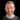Andrew McDonough

Please note: This page is imported from my wiki, which hasn't been updated in over 10 years. Some of the formatting was lost during the import. I'll try to get around to fixing it someday.

Excel

To view the object model, hit F2 while in the VBA editor.

Syntax

Declaring Variables

Dim mystring As String

Iterating over a Selection

Option 1 - When you only care that all cells are processed, and don’t care about their dimensions

E.g. To concatenate all cell values, display as a message box, and turn all processed cells red. Dim result As String For Each MyCell In Selection result = result & MyCell.Value MyCell.ColorIndex = Red Next MsgBox result

Option 2 - When you also care about the 2d structure of the cells

``````For RowCount = 1 To Selection.Rows.Count
thisdata = thisdata & "<tr>"
For ColCount = 1 To Selection.Columns.Count
If Selection.Cells(RowCount, ColCount).Text = ""
Next
Next``````

Message Box

A good way to debug Excel macros is to use a message box. This is similar to alert() in Javascript. MsgBox(“Here is my message”)

Functions

Creating

• Open the VBA editor (Tools -> Macros -> Visual Basic Editor)
• Create a new module (Insert -> Module)
• Write the functions

No Cell Object!

An experienced programmer may expect that the basic object in a work sheet is a Cell, with a Range being a collection of Cell objects. This is not the case. In fact a Cell object is simply a Range object, and it’s value can be determined by calling Range.Value If Range.Value is called on an actual Range, this returns the value of the first (top left) cell in the Range.

Iterating over named ranges

``````Dim msg As String
For Each Nm In ThisWorkbook.Names
msg = msg & "    " & Nm.Name
Next
MsgBox msg``````

Example Functions

Function five() five = 5; End Function

Joining strings by a delimiter

Function join(delimiter As String, r As Range) Dim result As String Dim thisDelimiter As String thisDelimiter = "" For Each c In r result = result + thisDelimiter + c.Value thisDelimiter = delimiter Next join = result End Function

Sentence Case

Function sc(r As Range) s = r.Value first = Left(s, 1) rest = Mid(s, 2, Len(s) - 1) sc = UCase(first) & LCase(rest) End Function

printf

The following is a simple function I wrote to simplify my continual dirty use of Excel’s CONCAT() function. It works in a similar way to C’s printf funtion where you pass it a template and a list of variables to substitute. The only placeholders used are strings (%s). This function is a good example of VBA’s ability to deal with an arbitrary number of arguments.

Function printf(s As String, ParamArray args()) For Each a In args s = Replace(s, “%s”, a, 1, 1) Next printf = s End Function

Example:

I use this function a lot to get Excel to write SQL for me: =printf(“INSERT INTO person values (‘%s’,‘%s’, ‘%s’);”, a1, b1, c1)“)

GetValue

Use this function to extract values of cells Function getValue(c) As Double getValue = Val(c) End Function

Use this function to extract the Hyperlink from a Cell in Excel Function getLink(r As Range) Dim result As String result = "" Dim link As Hyperlink If r.Hyperlinks.Count = 0 Then result = "" Else result = r.Hyperlinks(1).Address End If getLink = result End Function

TimeStamp

Writes a SQL formatted date to the current cell Sub InsertTimestamp() ActiveCell.FormulaR1C1 = “=TEXT(NOW(),""YYYY-MM-DD hh:mm"")” ActiveCell.Select Selection.Copy Selection.PasteSpecial Paste:=xlValues, Operation:=xlNone, SkipBlanks:= _ False, Transpose:=False End Sub